Study Materials

# NCERT Solutions for Class 9th Mathematics

Page 2 of 5

## Chapter 2. Polynomials

### Exercise 2.2

2. Polynomials

Exercise 2.2

Q1. Find the value of the polynomial 5x – 4x2 + 3 at

(i) x = 0 (ii) x = –1 (iii) x = 2

Solution:

(i) p(x) = 5x - 4x2 + 3

The value of the polynomial p(x) at x = 0 is given by

P(0) = 5(0) - 4(0)2 + 3

= 0 - 0 + 3

= 3

(ii) p(x) = 5x - 4x2 + 3

The value of the polynomial p(x) at x = 1 is given by

P(1) = 5(1) - 4(1)2 + 3

= 5 - 4 + 3

= 4

(iii) p(x) = 5x - 4x2 + 3

The value of the polynomial p(x) at x = 2 is given by

P(2) = 5(2) - 4(2)2 + 3

= 10 -16 + 3

= - 9

Q2.Find p(0), p(1) and p(2) for each of the following polynomials:

(i)  p(y) = y2 – y + 1

(ii) p(t) = 2 + t + 2t 2 – t 3

(iii) p(x) = x3

(iv) p(x) = (x – 1) (x + 1)

Solution:

(i) p(y) = y2 - y + 1

P(0) = (0)2- 0 + 1

=1

P(1) = (1)2- 1 + 1

= 1 - 1 + 1

= 1

P(2) = (2)2- 2 + 1 = 4 - 2 + 1 = 3

(ii) p(t) = 2 + t + 2t2- t3

P(0) = 2 + 0 + 2(0)2- (0)3

=2

P(1) = 2 + 1 + 2(1)2 - (1)3

= 4

P(2) = 2 + 2 + 2(2)2- (2)3

= 4 + 8 - 8

= 4

(iii) p(x) = x3

P(0)=(0)3 =0

P(1)=(1)3 =1

P(2)=(2)3=8

(iv)p(x) = (x – 1) (x + 1)

P(0)= (0-1) (0+1)=(-1) (1) =-1

P(1)= (1-1) (1+1) =0(1)  =0

P(2)= (2-1) (2+1)=1(3)  =3

Q3. Verify whether the following are zeroes of the polynomial, indicated against them.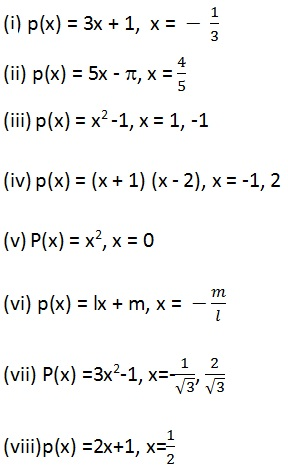Solution: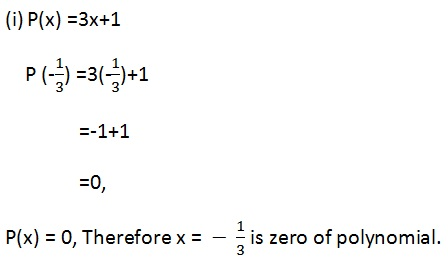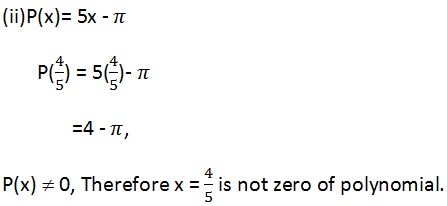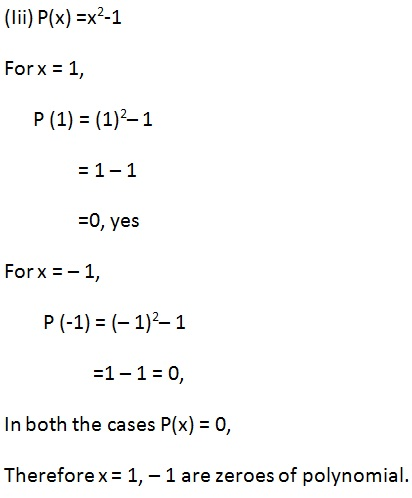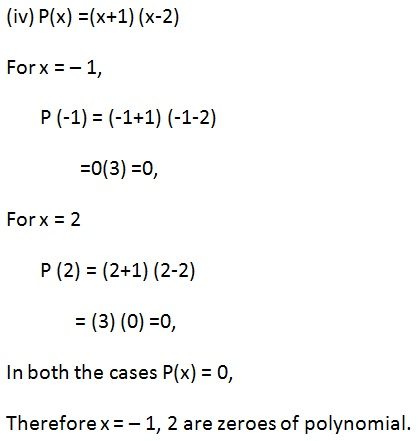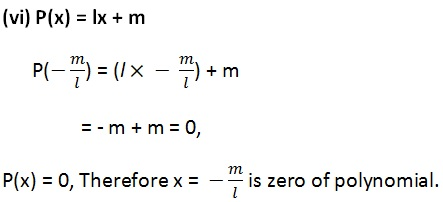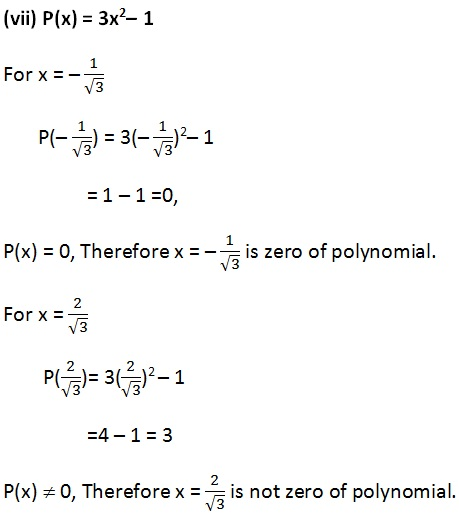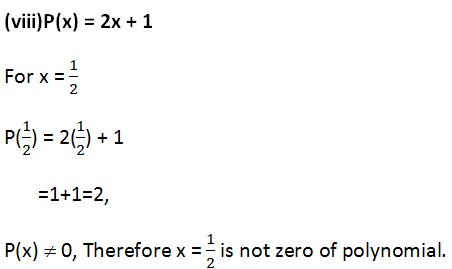Q4. Find the zero of the polynomial in each of the following cases:

(i)  P(x) = x + 5

(ii) P(x) = x 5

(iii) Px) = 2x + 5

(iv) P(x) = 3x 2

(v) P(x) = 3x

(vi) P(x) = ax, a  0

Solution (i) :

(i)   P(x) = x + 5

x + 5 = 0

⇒ x = - 5

The zero of the polynomial is - 5.

Solution (ii) :

(ii) P(x) = x  5

x  5  = 0

⇒ x = 5

The zero of the polynomial is  5.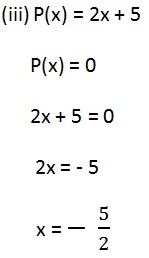The zero of the polynomial is - 5/2.

(iv)  P(x) = 3x - 2

3x - 2 = 0The zero of the polynomial is 2/3.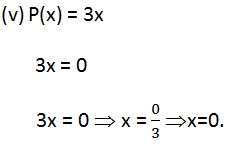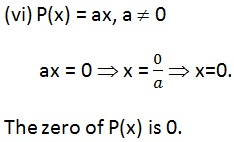Page 2 of 5

Chapter Contents: